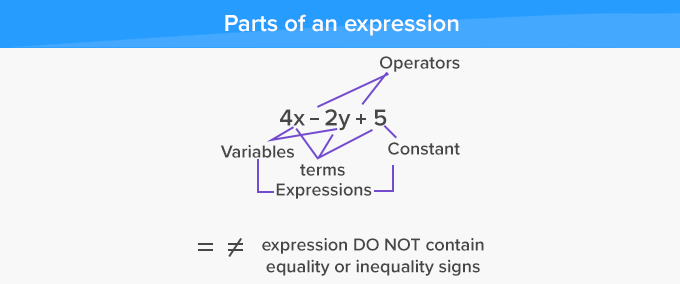# Numerical Expression - Definition with Examples

The Complete K-5 Math Learning Program Built for Your Child

• 40 Million Kids

Loved by kids and parent worldwide

• 50,000 Schools

Trusted by teachers across schools

• Comprehensive Curriculum

Aligned to Common Core

## What is Numerical Expression?

The term numerical expression is made up of two words, numerical meaning numbers, and expression meaning phrase. Thus, it is a phrase involving numbers.

A numerical expression in mathematics can be a combination of numbers, integers combined using mathematical operators such as addition, subtraction, multiplication, or division.

Examples of Numerical Expressions

We can form a numerical expression by combining numbers with various mathematical operators. There is no limit to the number of operators a numerical expression may contain. Some numerical expressions use only one operator between two numbers, and some may contain more.

Some examples of numerical expression are given below:

10 + 5

250 - 75

60 × 5 + 10

72 ÷ 8 × 5 - 4 + 1

82 + 4 - 10

## Writing Numerical expression

Any mathematical word problem is solved by first converting into a numerical expression. Below are some examples.

Candice has 10 chocolate bars. She gives 3 to her sister, 1 to her friend and eats 2 of them. Later she visits her grandmother, and she (grandmother) offers Candice 12 more chocolate bars. How many chocolate bars does Candice have now?

Let’s look at the numbers involved in the above problem. Candice starts with 10 bars, gives away 4 (3+1), eats 2 and then again gets 12 more from her grandmother. So, the numerical expression is

10 - 3 - 1 - 2 + 12

= 7 - 1 - 2 + 12

= 6 - 2 + 12

= 4 + 12

= 16

This gives us 16.

 Fun Facts A power is also a numerical expression that has two parts: an exponent and a base.An expression can also be a combination of variables and constants, combined using mathematical operations.  Such an expression is known as an algebraic expression.

For example:Won Numerous Awards & Honors